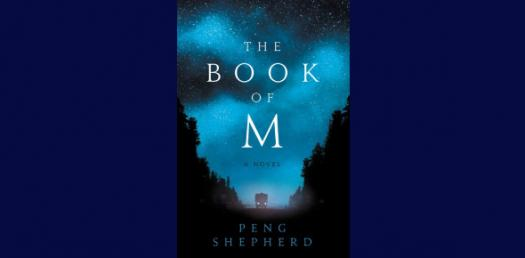Book M Ch 1 Pop Quiz

18 Questions | Total Attempts: 168Settings.

• 1.
Using this graph, write a short story about what is going on in the graph.  You can make up the characters and what they are doing BUT MAKE SURE THE STORY FOLLOWS WHAT THE GRAPH IS TELLING!  Also, make sure you tell me if this graph is showing speed or acceleration.
• 2.
Occurs when an object speeds up, slows down, or turns.
• A.

Inertia

• B.

Acceleration

• 3.
The measure of how difficult it is to stop a moving object.
• A.

Average speed

• B.

Momentum

• 4.
The speed and direction of a moving object
• A.

Velocity

• B.

Inertia

• 5.
The distance and direction from a starting point to a stopping point
• A.

Acceleration

• B.

Displacement

• 6.
A runner ran 200m in 140s. How fast were they traveling? Round to the nearest 10th. ________ m/s. (Put the numeric value only, not the m/s).
• 7.
A girl walked 200 meters north, 100 meters east,and 200 meters south. What is the total distance she traveled in meters? Put the numeric value only.
• 8.
A girl walked 200 meters north, 100 meters east,and 200 meters south. What was her displacement?  _____m East.
• 9.
An object has a speed of 2.00 m/s. Three seconds later it is going 8.00 m/s. What average acceleration did it experience?   ______ m/s 2.
• 10.
Find the acceleration of an object that speeds up to 52 m/s from 2.60 m/s in 4.8 s.  _____ m/s2.  Remember to round to the nearest 10th.
• 11.
What is the SI unit used for distance?
• A.

Kg

• B.

M

• 12.
What is the SI unit for mass?
• A.

Kg

• B.

M

• 13.
How is acceleration expressed?
• A.

M/s

• B.

M/s2

• 14.
A 50 kg object moves with a velocity of 10 m/s. What is its momentum?  _______ kg x m/s.  (Write the numeric value only).
• 15.
Momentum depends upon ____ and ____. Choose the two answers that belong in the blanks.
• A.

Mass

• B.

Volume

• C.

Velocity

• D.

Meters

• E.

Distance

• 16.
The formula for speed is
• A.

Final speed minus initial speed divided by time

• B.

Distance divided by time

• C.

Mass times velocity

• 17.
The formula for acceleration is
• A.

Final speed minus initial speed divided by time

• B.

Distance divided by time

• C.

Mass times velocity

• 18.
The formula for momentum is
• A.

Final speed minus initial speed divided by time

• B.

Distance divided by time

• C.

Mass times velocity

Related TopicsBack to top